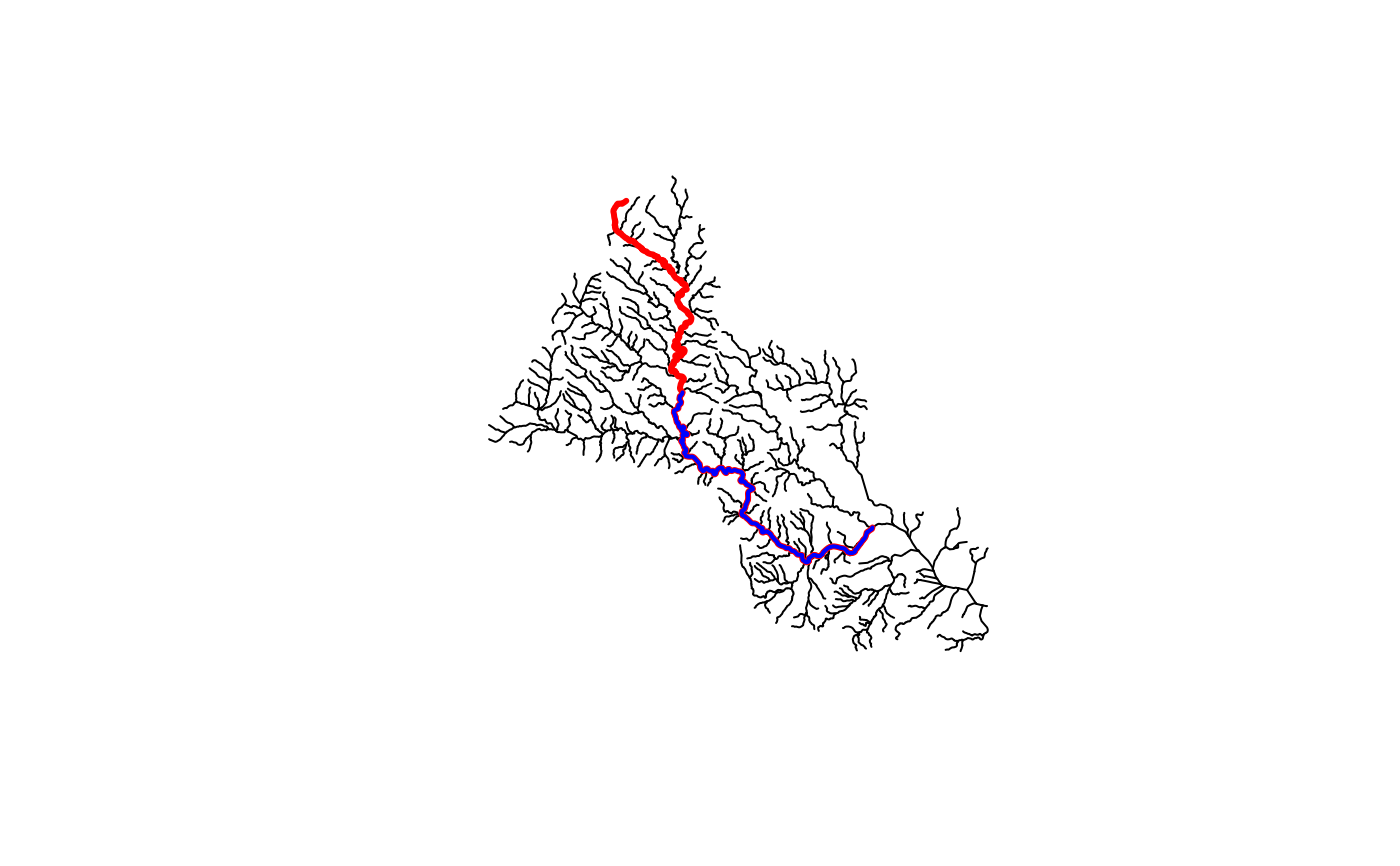Traverse NHDPlus network upstream main stem

get_UM(network, comid, distance = NULL, sort = FALSE, include = TRUE)

## Arguments

network

data.frame NHDPlus flowlines including at a minimum: COMID,Pathlength, LevelPathI, and Hydroseq.

comid

integer identifier to start navigating from.

distance

numeric distance in km to limit how many COMIDs are

sort

if TRUE, the returned COMID vector will be sorted in order of distance from the input COMID (nearest to farthest)

include

if TRUE, the input COMID will be included in the returned COMID vector returned. The COMID that exceeds the distance specified is returned.

## Value

integer vector of all COMIDs upstream of the starting COMID along the mainstem

## Examples

library(sf)

source(system.file("extdata", "sample_flines.R", package = "nhdplusTools"))

plot(sample_flines$geom) start_COMID <- 11690196 UM_COMIDs <- get_UM(sample_flines, start_COMID) plot(dplyr::filter(sample_flines, COMID %in% UM_COMIDs)$geom,
col = "red", add = TRUE, lwd = 3)

UM_COMIDs <- get_UM(sample_flines, start_COMID, distance = 50)
plot(dplyr::filter(sample_flines, COMID %in% UM_COMIDs)\$geom,
col = "blue", add = TRUE, lwd = 2)